Contemporary Mathematics

# 10.2Angles

Figure 10.21 This modern architectural design emphasizes sharp reflective angles as part of the aesthetic through the use of glass walls. (credit: “Société Générale @ La Défense @ Paris” by Images Guilhem Vellut/Flickr, CC BY 2.0)

### Learning Objectives

After completing this section, you should be able to:

1. Identify and express angles using proper notation.
2. Classify angles by their measurement.
3. Solve application problems involving angles.
4. Compute angles formed by transversals to parallel lines.
5. Solve application problems involving angles formed by parallel lines.

Unusual perspectives on architecture can reveal some extremely creative images. For example, aerial views of cities reveal some exciting and unexpected angles. Add reflections on glass or steel, lighting, and impressive textures, and the structure is a work of art. Understanding angles is critical to many fields, including engineering, architecture, landscaping, space planning, and so on. This is the topic of this section.

We begin our study of angles with a description of how angles are formed and how they are classified. An angle is the joining of two rays, which sweep out as the sides of the angle, with a common endpoint. The common endpoint is called the vertex. We will often need to refer to more than one vertex, so you will want to know the plural of vertex, which is vertices.

In Figure 10.22, let the ray $AB→AB→$ stay put. Rotate the second ray $AC→AC→$ in a counterclockwise direction to the size of the angle you want. The angle is formed by the amount of rotation of the second ray. When the ray $AC→AC→$ continues to rotate in a counterclockwise direction back to its original position coinciding with ray $AB→,AB→,$ the ray will have swept out $360∘.360∘.$ We call the rays the “sides” of the angle.

Figure 10.22 Vertex and Sides of an Angle

### Classifying Angles

Angles are measured in radians or degrees. For example, an angle that measures $ππ$ radians, or 3.14159 radians, is equal to the angle measuring $180∘.180∘.$ An angle measuring $π2π2$ radians, or 1.570796 radians, measures $90∘.90∘.$ To translate degrees to radians, we multiply the angle measure in degrees by $π180.π180.$ For example, to write $45∘45∘$ in radians, we have

$45∘(π180)=π4=0.785398radians.45∘(π180)=π4=0.785398radians.$

To translate radians to degrees, we multiply by $180π.180π.$ For example, to write $2π2π$ radians in degrees, we have

$2π(180π)=360∘.2π(180π)=360∘.$

Another example of translating radians to degrees and degrees to radians is $2π3.2π3.$ To write in degrees, we have $2π3(180π)=120∘.2π3(180π)=120∘.$ To write $30∘30∘$ in radians, we have $30∘(π180)=π630∘(π180)=π6$. However, we will use degrees throughout this chapter.

### FORMULA

To translate an angle measured in degrees to radians, multiply by $π180.π180.$

To translate an angle measured in radians to degrees, multiply by $180π.180π.$

Several angles are referred to so often that they have been given special names. A straight angle measures $180∘180∘$; a right angle measures $90∘;90∘;$ an acute angle is any angle whose measure is less than $90∘;90∘;$ and an obtuse angle is any angle whose measure is between $90∘90∘$ and $180∘.180∘.$ See Figure 10.23.

Figure 10.23 Classifying and Naming Angles

An easy way to measure angles is with a protractor (Figure 10.24). A protractor is a very handy little tool, usually made of transparent plastic, like the one shown here.

Figure 10.24 Protractor (credit: modification of work “School drawing tools” by Marco Verch/Flickr, CC BY 2.0)

With a protractor, you line up the straight bottom with the horizontal straight line of the angle. Be sure to have the center hole lined up with the vertex of the angle. Then, look for the mark on the protractor where the second ray lines up. As you can see from the image, the degrees are marked off. Where the second ray lines up is the measurement of the angle.

### Checkpoint

Make sure you correctly match the center mark of the protractor with the vertex of the angle to be measured. Otherwise, you will not get the correct measurement. Also, keep the protractor in a vertical position.

### Notation

Naming angles can be done in couple of ways. We can name the angle by three points, one point on each of the sides and the vertex point in the middle, or we can name it by the vertex point alone. Also, we can use the symbols $∠∠$ or $∡∡$ before the points. When we are referring to the measure of the angle, we use the symbol $m∡m∡$. See Figure 10.25.

Figure 10.25 Naming an Angle

We can name this angle $∡BAC∡BAC$, or $∡CAB∡CAB$, or $∡A.∡A.$

### Example 10.7

#### Classifying Angles

Determine which angles are acute, right, obtuse, or straight on the graph (Figure 10.26). You may want to use a protractor for this one.

Figure 10.26

1.
Determine which angles are acute, obtuse, right, and straight in the graph.

Two angles with the same starting point or vertex and one common side are called adjacent angles. In Figure 10.27, angle $∠DBC∠DBC$ is adjacent to $∠CBA∠CBA$. Notice that the way we designate an angle is with a point on each of its two sides and the vertex in the middle.

### Supplementary Angles

Two angles are supplementary if the sum of their measures equals $180∘.180∘.$ In Figure 10.28, we are given that $m∡FBE=35∘,m∡FBE=35∘,$ so what is $m∡ABE?m∡ABE?$ These are supplementary angles. Therefore, because $m∡ABF=180∘m∡ABF=180∘$, and as $180∘−35∘=145∘,180∘−35∘=145∘,$ we have $m∡ABE=145∘.m∡ABE=145∘.$

Figure 10.28 Supplementary Angles

### Example 10.8

#### Solving for Angle Measurements and Supplementary Angles

Solve for the angle measurements in Figure 10.29.

Figure 10.29

1.
Solve for the angle measurements in the figure shown.

### Complementary Angles

Two angles are complementary if the sum of their measures equals $90∘.90∘.$ In Figure 10.30, we have $m∡ABC=30∘,m∡ABC=30∘,$ and $m∡ABD=90∘.m∡ABD=90∘.$ What is the $m∡CBD?m∡CBD?$ These are complementary angles. Therefore, because $90∘−30∘=60∘,90∘−30∘=60∘,$ the $∡CBD=60°.∡CBD=60°.$

Figure 10.30 Complementary Angles

### Example 10.9

#### Solving for Angle Measurements and Complementary Angles

Solve for the angle measurements in Figure 10.31.

Figure 10.31

1.
Find the measure of each angle in the illustration.

### Vertical Angles

When two lines intersect, the opposite angles are called vertical angles, and vertical angles have equal measure. For example, Figure 10.32 shows two straight lines intersecting each other. One set of opposite angles shows angle markers; those angles have the same measure. The other two opposite angles have the same measure as well.

Figure 10.32 Vertical Angles

### Example 10.10

#### Calculating Vertical Angles

In Figure 10.33, one angle measures $40∘.40∘.$ Find the measures of the remaining angles.

Figure 10.33

1.
Given the two intersecting lines in the figure shown and $m\measuredangle 2 = {67^ \circ },$ find the measure of the remaining angles.

### Transversals

When two parallel lines are crossed by a straight line or transversal, eight angles are formed, including alternate interior angles, alternate exterior angles, corresponding angles, vertical angles, and supplementary angles. See Figure 10.34. Angles 1, 2, 7, and 8 are called exterior angles, and angles 3, 4, 5, and 6 are called interior angles.

Figure 10.34 Transversal

### Alternate Interior Angles

Alternate interior angles are the interior angles on opposite sides of the transversal. These two angles have the same measure. For example, $∡3∡3$ and $∡6∡6$ are alternate interior angles and have equal measure; $∡4∡4$ and $∡5∡5$ are alternate interior angles and have equal measure as well. See Figure 10.35.

Figure 10.35 Alternate Interior Angles

### Alternate Exterior Angles

Alternate exterior angles are exterior angles on opposite sides of the transversal and have the same measure. For example, in Figure 10.36, $∡2∡2$ and $∡7∡7$ are alternate exterior angles and have equal measures; $∡1∡1$ and $∡8∡8$ are alternate exterior angles and have equal measures as well.

Figure 10.36 Alternate Exterior Angles

### Corresponding Angles

Corresponding angles refer to one exterior angle and one interior angle on the same side as the transversal, which have equal measures. In Figure 10.37, $∡1∡1$ and $∡5∡5$ are corresponding angles and have equal measures; $∡3∡3$ and $∡7∡7$ are corresponding angles and have equal measures; $∡2∡2$ and $∡6∡6$ are corresponding angles and have equal measures; $∡4∡4$ and $∡8∡8$ are corresponding angles and have equal measures as well.

Figure 10.37 Corresponding Angles

### Example 10.11

#### Evaluating Space

You live on the corner of First Avenue and Linton Street. You want to plant a garden in the far corner of your property (Figure 10.38) and fence off the area. However, the corner of your property does not form the traditional right angle. You learned from the city that the streets cross at an angle equal to $150∘.150∘.$ What is the measure of the angle that will border your garden?

Figure 10.38

1.
Suppose you have a similar property to the one in Figure 10.53, but the angle that corresponds to the garden corner is ${50^ \circ }$. What is the measure between the two cross streets?

### Example 10.12

#### Determining Angles Formed by a Transversal

In Figure 10.39 given that angle 3 measures $40∘,40∘,$ find the measures of the remaining angles and give a reason for your solution.

Figure 10.39

1.
In the given figure if $m\measuredangle 1 = {120^ \circ }$, find the $m\measuredangle 5$, $m\measuredangle 4$, and $m\measuredangle 8$.

### Example 10.13

#### Measuring Angles Formed by a Transversal

In Figure 10.40 given that angle 2 measures $23∘,23∘,$ find the measure of the remaining angles and state the reason for your solution.

Figure 10.40

1.
In the provided figure given that the $m\measuredangle 2 = {48^ \circ }$, find $m\measuredangle 1$, and $m\measuredangle 5.$

### Example 10.14

#### Finding Missing Angles

Find the measures of the angles 1, 2, 4, 11, 12, and 14 in Figure 10.41 and the reason for your answer given that $l1l1$ and $l2l2$ are parallel.

Figure 10.41

1.
Using Figure 10.58, find the measures of angles 5, 6, 7, 8, and 9.

### Who Knew?

#### The Number 360

Did you ever wonder why there are $360∘360∘$ in a circle? Why not $100∘100∘$ or $500∘?500∘?$ The number 360 was chosen by Babylonian astronomers before the ancient Greeks as the number to represent how many degrees in one complete rotation around a circle. It is said that they chose 360 for a couple of reasons: It is close to the number of days in a year, and 360 is divisible by 2, 3, 4, 5, 6, 8, 9, 10, …

Classify the following angles as acute, right, obtuse, or straight.
10.
$m\measuredangle = {180^ \circ }$
11.
$m\measuredangle = {176^ \circ }$
12.
$m\measuredangle = {90^ \circ }$
13.
$m\measuredangle = {37^ \circ }$
For the following exercises, determine the measure of the angles in the given figure.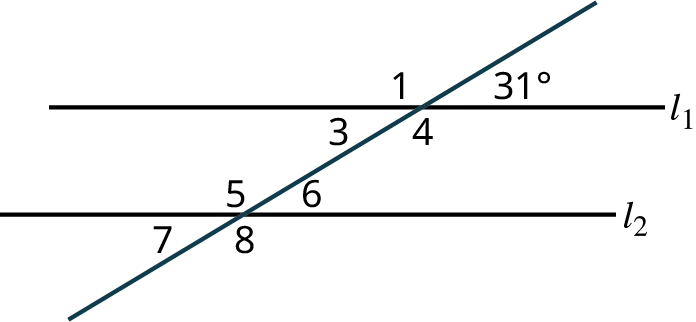14.
Find the measure of $\measuredangle 1$ and state the reason for your solution.
15.
Find the measure of $\measuredangle 3$ and state the reason for your solution.
16.
Find the measure of $\measuredangle 5$ and state the reason for your solution.

### Section 10.2 Exercises

Classify the angles in the following exercises as acute, obtuse, right, or straight.
1 .
$m\measuredangle = {32^ \circ }$
2 .
$m\measuredangle = {120^ \circ }$
3 .
$m\measuredangle = {180^ \circ }$
4 .
$m\measuredangle = {90^ \circ }$
5 .
$m\measuredangle = {110^ \circ }$
6 .
$m\measuredangle = {45^ \circ }$
7 .
Use the given figure to solve for the angle measurements.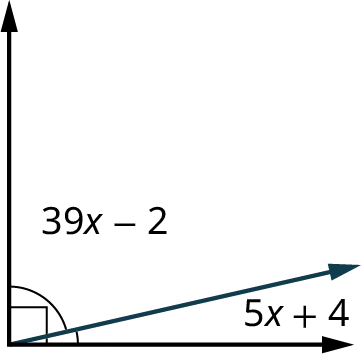8 .
Use the given figure to solve for the angle measurements.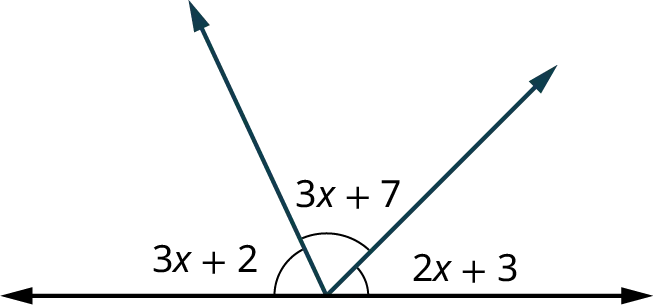9 .
Give the measure of the supplement to ${89^ \circ }.$
Use the given figure for the following exercises. Let angle 2 measure ${35^ \circ }$.10 .
Find the measure of angle 1 and state the reason for your solution.
11 .
Find the measure of angle 3 and state the reason for your solution.
12 .
Find the measure of angle 4 and state the reason for your solution.
13 .
Find the measure of angle 5 and state the reason for your solution.
14 .
Find the measure of angle 6 and state the reason and state the reason for your solution.
15 .
Find the measure of angle 7 and state the reason for your solution.
16 .
Find the measure of angle 8 and state the reason for your solution.
17 .
Use the given figure to solve for the angle measurements.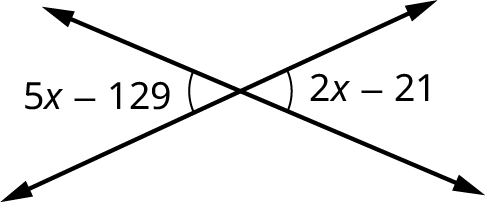Use the given figure for the following exercises.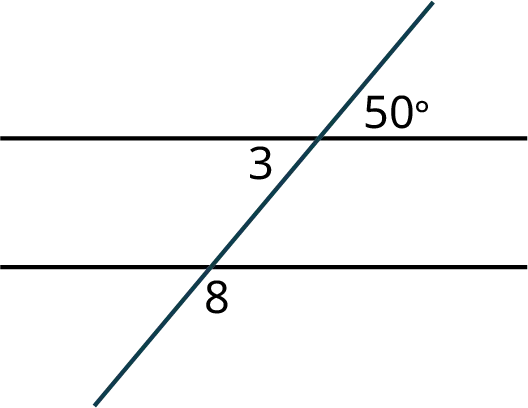18 .
Find the measure of angle 3 and explain the reason for your solution.
19 .
Find the measure of angle 8 and explain the reason for your solution.
Order a print copy

As an Amazon Associate we earn from qualifying purchases.